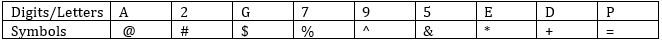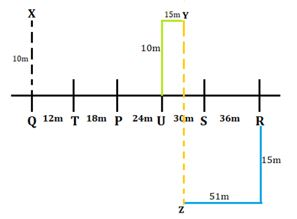Latest Banking jobs   »

# Reasoning Quizzes For IBPS RRB PO/Clerk Mains 2022- 17th September

Direction (1-5): Study the following information carefully and answer the questions given below:
Six Horse i.e., P, Q, R, S, T and U are standing in a row facing north at a distance which is a successive multiple of 6 in an increasing order from the left to right.
Horse S is standing second from one of the extreme ends. Only two horses stand between the S and T. Two horses stand between R and P who does not stand adjacent to S. U stands right of Q, who does not stand next to P. Neither R nor S is not an immediate neighbour of Q. Total distance between the horse P and Horse S is 54. Now, horse U is moving towards North direction and after going 10m, it turns to right and goes 15m to reach at point Y. Horse R is moving towards South direction and after going 15m it takes right turn and goes 51m to reach at point Z.

Q1. What is the shortest distance between Point Y and point Z?
(a) 20m
(b) 25m
(c) 18m
(d) 30m
(e) None of these

Q2. In which direction point Y is with respect to point Z?
(a) North
(b) North west
(c) North east
(d) South west
(e) None of these

Q3. What is the total distance between Horse S and Horse T?
(a) 54m
(b) 80m
(c) 72m
(d) 36m
(e) None of these

Q4. If Horse Q moves 10m in north to reach point X, then what is the shortest distance between point X and point Y?
(a) 54m
(b) 69m
(c) 47m
(d) 65m
(e) None of these

Q5. What is total distance between horse Q and Horse R?
(a) 106m
(b) 96m
(c) 120m
(d) 118m
(e) None of these

Directions (6-9): In each of the questions given below, a group of digits is given followed by four combinations of symbols numbered (a), (b), (c) and (d). You have to find out which of the four combinations correctly represents the group of digits based on the symbol codes and the conditions given below. If none of the four combinations represents the group of digits correctly, give (e) ‘None of these’ as the answer.Conditions for coding-
(1) If the first element is even number and the last element is vowel then both are to be coded as the code of vowel.
(2) If the first element is odd number and last element is consonant then both are to be coded as the code of the last element.
(3) If the first element is vowel and the last element is consonant then the codes of both elements are to be interchanged.
(4) If the first element is consonant and last element is even number then both are to be coded as the code of the last element.

Q6. DEG7A2
(a) #*\$%@#
(b) +*\$%@+
(c) #*\$@%#
(d) #*\$%@+
(e) None

Q7. 9DE52P
(a) =*+&#=
(b) ^+*&#^
(c) =+*&#=
(d) =+*#&^
(e) None

Q8. 2G795A
(a) @\$^%&@
(b) @\$%^&@
(c) #\$%^&#
(d) @4%^&#
(e) None

Q9. E2PD9G
(a) *#=+^\$
(b)\$ #=+^\$
(c)* #=+^*
(d) \$#=+^*
(e) None

Directions (10): In the given question, a statement is given below followed by two conclusions numbered I and II. You have to assume everything in the statement to be true, then consider the two conclusions together and decide which of them logically follows beyond a reasonable doubt from the information given in the statement.

Q10. Statement: Irregularity is a cause for failure in exams.
Some regular students fail in the examinations.
Conclusions: I. All failed students are regular.
II. All successful students are not regular.
(a) if only conclusion I follows;
(b) if only conclusion II follows;
(c) If either I or II follows;
(d) if neither I nor II follows; and
(e) if both I and II follow.

Directions (11-15): A word and number arrangement machine when given an input line of words and numbers rearranges them following a particular rule in each step. The following is an illustration of an input and rearrangement.

Input: 56 set 90 colour 43 pink 65 ago toy 17 94 near
Step I: 19 56 set 90 colour 43 pink 65 toy 94 near ago
Step II: colour 19 56 set 90 pink 65 toy 94 near ago 45
Step III: 54 colour 19 set 90 pink 65 toy 94 ago 45 near
Step IV: pink 54 colour 19 set 90 toy 94 ago 45 near 67
Step V: 88 pink 54 colour 19 toy 94 ago 45 near 67 set
Step VI: toy 88 pink 54 colour 19 ago 45 near 67 set 92

And Step VI is the last step of the rearrangement of the above input.
As per the rules followed in the above steps, find out in each of the following questions the appropriate step for the given input.

Input: 50 hot 84 begin 37 economy 59 again new 11 88 cool

Q11. In which step the elements ‘13 new 88’ found in the same order?
(a) Step I
(b) Step II
(c) Step III
(d) Step V
(e)Step VI

Q12. In step IV, which of the following word/number would be at 2nd position to the left of 7th from the right end?
(a) 13
(b) hot
(c) 84
(d) new
(e) 88

Q13. How many steps required for completing the above arrangement?
(a) Three
(b) Four
(c) Six
(d) Seven
(e) Five

Q14. Which of the following would be the step III after arrangement?
(a) 48 begin 13 hot 84 economy 59 new 88 again 39 cool
(b) 48 begin 13 hot 80 economy 57 new 86 again 37 cool
(c) 48 begin 15 hot 82 economy 57 new 86 again 37 cool
(d) 48 begin 13 hot 82 economy 57 new 86 again 37 cool
(e) None of these

Q15. In step VI, ‘82’ is related to ‘new’ and ‘13’ is related to ‘begin’. In the same way ‘61’ is related to?
(a) cool
(b) new
(c) economy
(d) hot
(e) None of these

Solutions

Solution (1-5):
Sol.S1. Ans. (b)
S2. Ans. (a)
S3. Ans. (c)
S4. Ans. (b)
S5. Ans. (c)

S6. Ans.(a)
Sol. Here, condition (4) is used

S7. Ans.(c)
Sol. Here, condition (2) is used

S8. Ans.(b)
Sol. Here, condition (1) is used

S9. Ans.(d)
Sol. Here, condition (3) is used

S10. Ans. (d)
Sol. The-given statement clearly implies that all irregular and some regular students fail in the examinations. This, in turn, means that all successful students are regular but not all regular students are successful. So, neither I nor II follows.

Direction (11-15):
Sol. Students let us understand the Logic behind this Question and let’s understand how to solve it. When we see in each step, then we can find that there are both number and words are arranged in each step.

(1) For words arrangement- Words are arranged according to ascending order given in English alphabetical order. In first step the words which comes first according to English dictionary arranged first to extreme right. And in second step next word are arranged to extreme left.
And this process is continued in further step.
(2) For number arrangement- Number are arranged according to ascending order. In first step lowest number is arranged on extreme left. And in second step next number is arranged on extreme right. And this process is continued in further step (Each odd number is added by two (+2) while they are arranged and two is subtracted from each even number (-2) while they are arranged).

Input: 50 hot 84 begin 37 economy 59 again new 11 88 cool
Step I: 13 50 hot 84 begin 37 economy 59 new 88 cool again
Step II: begin 13 50 hot 84 economy 59 new 88 cool again 39
Step III: 48 begin 13 hot 84 economy 59 new 88 again 39 cool
Step IV: economy 48 begin 13 hot 84 new 88 again 39 cool 61
Step V: 82 economy 48 begin 13 new 88 again 39 cool 61 hot
Step VI: new 82 economy 48 begin 13 again 39 cool 61 hot 86

S11. Ans. (d)
S12. Ans. (a)
S13. Ans.(c)
S14. Ans.(a)
S15. Ans. (a)#### Congratulations!Download Hindu Review of October 2021: Free PDF